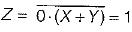Courses

# Test: Logic Gates & Switching Circuits- 2

## 20 Questions MCQ Test Topicwise Question Bank for Electrical Engineering | Test: Logic Gates & Switching Circuits- 2

Description
This mock test of Test: Logic Gates & Switching Circuits- 2 for Electrical Engineering (EE) helps you for every Electrical Engineering (EE) entrance exam. This contains 20 Multiple Choice Questions for Electrical Engineering (EE) Test: Logic Gates & Switching Circuits- 2 (mcq) to study with solutions a complete question bank. The solved questions answers in this Test: Logic Gates & Switching Circuits- 2 quiz give you a good mix of easy questions and tough questions. Electrical Engineering (EE) students definitely take this Test: Logic Gates & Switching Circuits- 2 exercise for a better result in the exam. You can find other Test: Logic Gates & Switching Circuits- 2 extra questions, long questions & short questions for Electrical Engineering (EE) on EduRev as well by searching above.
QUESTION: 1

### For the logic diagram shown below, the output f is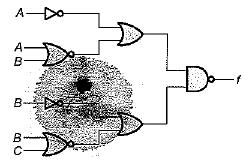Solution:

From given logic diagram, output is: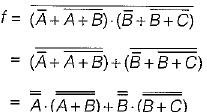= A.(A + B) + B.(B + C)
= A.A + A B + BB + BC
= A + AB + B + BC
= A(1 + B) + B(1 + C)
= A + B

QUESTION: 2

### The minimum number of NOR gates requires to implement the expression f(A, B, C) = πM(0, 1, 2, 3, 4, 7) is

Solution:

Given expressio n is f = πM( 0, 1, 2, 3, 4, 7)
The K-map for this expression is shown below.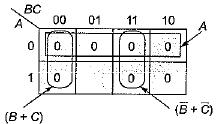The minimized expression in POS form is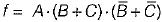The minimized expression 'f' can be implemented using two input NOR gate as shown below.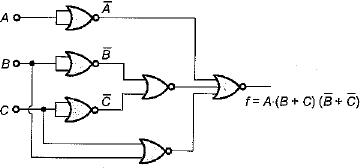Thus, we require six NOR gates.

QUESTION: 3

### Consider the following statements associated with logic gates: 1. An OR gate in the positive logic system becomes an AND gate in the negative logic system and vice-versa. 2. The dual of an expression is obtained by changing ANDs to ORs, ORs to ANDs.Os to 1s, to Os and by complementing the variables. 3. NAND and NOR are the only two available universal logic gates. 4. Universal gates do not follow commutative law 5. On logic diagrams, an inversion bubble at the point where the input is connected to a gate, indicates an active-HIGH input. Which of the statements given above is/are not correct?

Solution:
• Statement-1 is correct because positive and negative logics give rise to a basic duality in all Boolean identities. Here, an AND gate becomes an OR gate and an OR gate becomes an AND gate and vice-versa.
• For obtaining dual expression, variables are not complemented. Hence, statement-2 is not correct.
• NAND and NOR are the only two universal gates while NOT, OR, AND are three basic gates. Thus, statement-3 is correct.
• Universal gates follow associate law but not commutative law. Hence, statement-4 is correct.
• An inversion bubble on logic diagram at input indicates an active-LOW input. Thus, statement-5 is not correct.

Hence, statements 1 and 3 are correct while statements 2, 4 and 5 are false.

QUESTION: 4

The number of two input NAND gate required to implement an OR gate and an EX-NOR gate are respectively

Solution:

OR gate using NAND gate: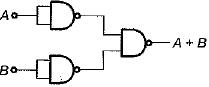EX-NOR gate using NAND gate: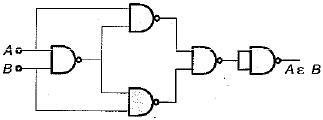Thus, we require 3 NAND gates for an OR gate while 5 NAND gates for an EX-NOR gate.

QUESTION: 5

Three set of simultaneous equations are given by
(i)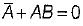(ii) AB = AC
(iii)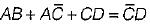The solution of the above three set of simultaneous equations will be

Solution:

Given,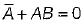or,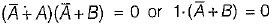i.e.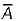must be 0 and B must be 0 Hence, A = 1 and B = 0
Also, AB = AC or 1.B - 1.C
or B = C
Hence, C = 0 (∵ B = 0)
Also,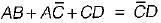or, 1.0 + 1.1 + 0.D = 1.D
or D = 1
Thus, the values of A, 6, Cand Dare given by
A = 1, B = 0, C = 0 and D = 1.

QUESTION: 6

If one of the input to an EX-NOR gate is fixed to logic ‘1’, then output of this gate will be

Solution: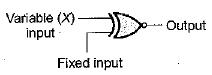Output = X.1 +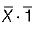= X = Input

QUESTION: 7

An AND gate

Solution: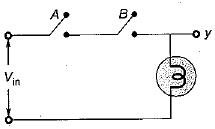The truth table for above circuit is shown below.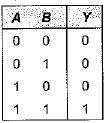Hence, the output y is equivalent to output of an AND gate having two inputs A and 6. Thus, an AND gate is equivalent to a series switching circuit.

QUESTION: 8

An XOR gate produces output 1 only when two inputs are

Solution:

For same inputs, A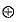A = 0
For different inputs,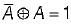QUESTION: 9

Consider the truth table shown beiow: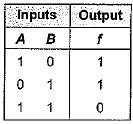The logic gate represented by the above truth table is

Solution:

For same inputs, output (f) = 0
For different inputs, output (f) = 1
Hence, given truth table represents an EX-OR gate.

QUESTION: 10

A logic gate is an electronic circuit which

Solution:
QUESTION: 11

The output of a logic gate is ‘0’ when both its inputs are different. The gate is

Solution:

Truth tables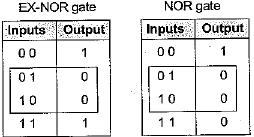Thus, the gate can be either an EX-NOR or a NOR gate.

QUESTION: 12

For the diode circuit shown below, both the diodes D1, and Dare ideal.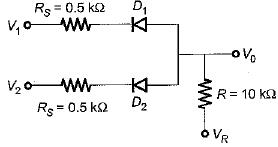If VR= +5 V, then match List-I (Values of V1 and V2) with List-Il (Output voltage V0) and select the correct answer using the codes given below the lists:
List-I
V1 = 0 and V2 = 5 volt
V= V2 = 5 volt
V1 = V2 = 0 volt
List-ll
1 . 5 volt
2. 0.12 volt
3. 0.24 volt
4. 2.5 volt
Codes:
A B C
(a) 3 1 2
(b) 4 1 3
(c) 2 3 4
(d) 3 4 2

Solution:
• When V= 0 volt and V2 = 5 volt, diode D1 will be on.
Using voltage division rule,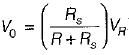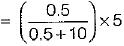= 0.24 volt
• When V1 = V2 = 5 volt, then both diodes are off, no current flows anywhere, and V0 = 5 volt.
• When V1 = V2 = 0 volt, then both diodes D1 and D2 will be on and their two Rs's will effectively come in parallel.

Thus,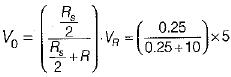= 0.12 V

QUESTION: 13

A circuit has three inputs and one output. The output is 1 if at least two of the three input variables are 1, otherwise it is zero. The minimum number of basic gates required to implement the output (Y) are

Solution:

The truth table for the given condition is shown below: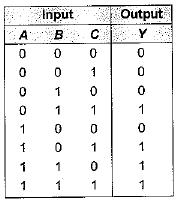The K-map for above truth table is shown below.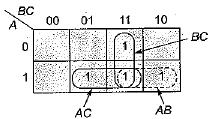Thus, Y = AB + BC + CA which can be implemented using 3 AND gates and 1 OR gate (total 4 basic gates).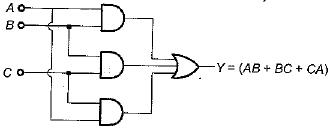QUESTION: 14

The boolean expression for the output f of the digital circuit shown below is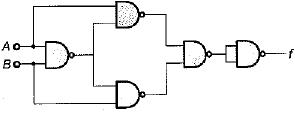Solution:

Let the output of second last NAND gate be X.
Then,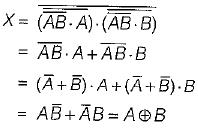Thus,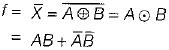Given circuit represents EX-NOR gate using NAND gate

QUESTION: 15

In the truth table of an N-input OR gate, in the column for the output of the gate,

Solution:

A two input OR gate truth table is shown below: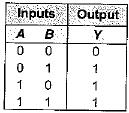From the truth table of OR gate it is clear tha output is 0’s only when all the inputs are 0’s otherwise for all other input combination outpu is always 1's. Hence, in the column for the outpu of the OR gate, the number of zeros is always 1's.

QUESTION: 16

Consider the following statements associated with logic gates:
1. Logic circuit of any complexity can be realised by using only the three basic gates namely AND, GR and NOT.
2. AND, OR and NOT .gates are called universal building blocks.
3. AND/OR/INVERT logic (AO! logic) can be converted to NAND logic or NOR logic.
4. A NAND gate can be used as an inverter by connecting all its input terminals except one, to logic 1 and applying the signal to be inverted to the remaining terminal.
Q. Which of the statements given above is/are correct?

Solution:
• Statement-1 is correct because any circuit can be realized using AND, OR and NOT gates.
• The two logic gates NAND and NOR can realize any logic circuit single-handedly. Hence, these two gates are called universal building blocks. AND, OR and NOT are called basic gates. Thus, statement-2 is not correct.
• Both NAND and NOR gates can perform all the three basic logic functions (AND, OR and NOT). Therefore, AND/OR/INVERT logic can be converted to NAND logic or NOR logic. Hence, statement-3 is correct.
•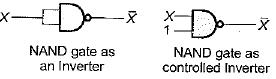Statement-4 is not correct.
QUESTION: 17

Assertion (A): When a number of variables are to be X-NORed, a number of two-input X-NOR gates can be used.
Reason (R): Three or more variable X-NOR gates will make the circuit costly.

Solution:

Since three or more variable X-NOR gates do not exist, therefore when a number of variables are to be X-NORed, a number of two input X-NOR gases have to be used. Thus, reason is not a correct statement.

QUESTION: 18

If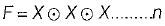times, then the value of F is

Solution:

When n is even,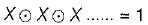When n is odd,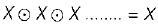QUESTION: 19

The simplified version of the logic circuit shown below consists of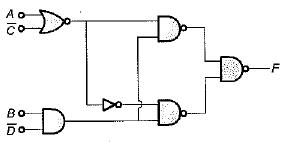Solution:

From the given circuit, output is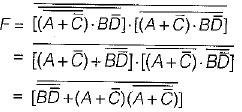(∴ (X + Y) (X + Z) = X + YZ)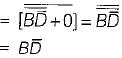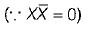Therefore, the given logic circuit can be simplified as Do shown below.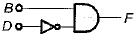Thus, it require a AND gate and a NOT gate.

QUESTION: 20

The circuit shown in figure is a ECL OR-AND- INVERTER circuit. The output Z is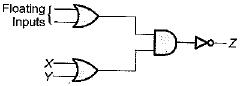Solution:

In case of ECL circuit, the floating inputs are considered as logic ‘0’. Hence output